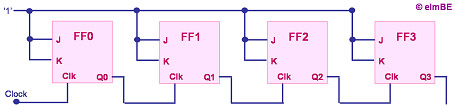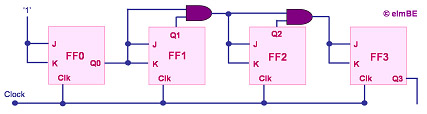Digital Counters

A digital counter, or simply counter, is a semiconductor device that is used for counting the number of times that a digital event has occurred.  The counter's output is indexed by one LSB every time the counter is clocked.

A simple implementation of a 4-bit counter is shown in Figure 1, which consists of 4 stages of cascaded J-K flip-flops. This is a binary counter, since the output is in binary system format, i.e., only two digits are used to represent the count, i.e., '1' and '0'.  With only 4 bits, it can only count up to '1111', or decimal number 15.

As one can see from Figure 1, the J and K inputs of all the flip-flops are tied to '1', so that they will toggle between states every time they are clocked. Also, the output of each flip-flop in the counter is used to clock the next flip-flop.  As a result, the succeeding flip-flop toggles between '1' and '0' at only half the frequency as the flip-flop before it.Figure 1. A Simple Ripple Counter Consisting of J-K Flip-flops

Thus, in Figure 1's 4-bit example, the last flip-flop will only toggle after the first flip-flop has already toggled 8 times. This type of binary counter is known as a 'serial', 'ripple', or 'asynchronous' counter.  The name 'asynchronous' comes from the fact that this counter's flip-flops are not being clocked at the same time.

A 4-bit counter, which has 16 unique states that it can count through, is also called a modulo-16 counter, or mod-16 counter.  By definition, a modulo-k or base-k counter is one that returns to its initial state after k cycles of the input waveform.  A counter that has N flip-flops is a modulo 2N counter.

An asynchronous counter has a serious drawback - its speed is limited by the cumulative propagation times of the cascaded flip-flops.  A counter that has N flip-flops, each of which has a propagation time t, must therefore wait for a duration equal to N x t before it can undergo another transition clocking.

A better counter, therefore, is one whose flip-flops are clocked at the same time. Such a counter is known as a synchronous counter.  A simple 4-bit synchronous counter is shown in Figure 2.

Not all counters with N flip-flops are designed to go through all its 2N possible states of count.  In fact, digital counters can be used to output decimal numbers by using logic gates to force them to reset when the output becomes equal to decimal 10. Counters used in this manner are said to be in binary-coded decimal (BCD).Figure 2.  A Simple Synchronous Counter Consisting of J-K Flip-flops and AND gates